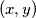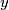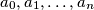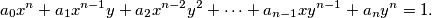### IMO Shortlist 2017 problem N7

Kvaliteta:
Avg: 4.0
Težina:
Avg: 9.0

An ordered pair$(x, y)$ of integers is a primitive point if the greatest common divisor of$x$ and$y$ is$1$. Given a finite set$S$ of primitive points, prove that there exist a positive integer$n$ and integers$a_0, a_1, \ldots , a_n$ such that, for each$(x, y)$ in$S$, we have:Source: https://www.imo-official.org/problems/IMO2017SL.pdf